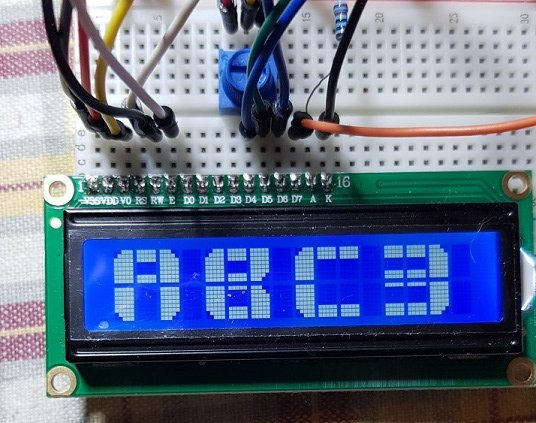B4R Tutorial Big/Large Alphanumeric Characters(LCD1602)

Discussion in 'B4R Tutorials' started by Johan Schoeman, Jun 8, 2019.

1. The attached project comes from here. Used Inline C to create and display the big/large alphanumeric characters.

I am sure you will figure out how to make use of it (i.e calling and display other alphanumeric characters other than what the attached B4R project is displaying).

You need to select/enable the rLiquidCrystal library in the B4R IDE.
Wiring diagram is the same as in this posting:
https://www.b4x.com/android/forum/t...ar-graph-on-your-lcd-display-lcd-1602.106552/Sample Code (change it to your liking):
Code:
'LCD Display - LCD 1602
'See attached wiring diagram

#Region Project Attributes

#AutoFlushLogs: True

#CheckArrayBounds: True

#StackBufferSize: 300
#End Region

Sub Process_Globals

'These global variables will be declared once when the application starts.
'Public variables can be accessed from all modules.
Public Serial1 As Serial

Dim lcd As LiquidCrystal

Dim rw As Int

Dim cln As Int

End Sub

Private Sub AppStart
Serial1.Initialize(
115200)

Log("AppStart")

lcd.Initialize(
8,255,9Array As Byte(4,5,6,7))         'using pins 4, 5, 6, 7 for data, pin 8 = RS, pin 9 = Enable
Delay(10)
lcd.Begin(
162)                                        'set LCD display for 16 columns and 2 rows
lcd.Clear

RunNative(
"createLT",Null)
RunNative(
"createUB",Null)
RunNative(
"createRT",Null)
RunNative(
"createLL",Null)
RunNative(
"createLB",Null)
RunNative(
"createLR",Null)
RunNative(
"createUMB",Null)
RunNative(
"createLMB",Null)

rw =
0
cln =
0
RunNative(
"custom1"Null)               'write custom A - it takes up 3 columns and 2 rows (starting at column = 0 and row = 0)

Delay(
1)
rw =
0
cln =
4
RunNative(
"custom4"Null)               'write custom B - it takes up 3 columns and 2 rows (starting at column = 4 and row = 0)

Delay(
1)
rw =
0
cln =
8
RunNative(
"custom7"Null)               'write custom C - it takes up 3 columns and 2 rows (starting at column = 8 and row = 0)

Delay(
1)
rw =
0
cln =
12
RunNative(
"custom9"Null)               'write custom 3 - it takes up 3 columns and 2 rows (starting at column = 12 and row = 0)

End Sub

'the C code below creates the custom chars and displays them
#if C

// the 8 arrays that form each segment of the custom numbers
byte LT =
{
B00111,
B01111,
B11111,
B11111,
B11111,
B11111,
B11111,
B11111
};
byte UB =
{
B11111,
B11111,
B11111,
B00000,
B00000,
B00000,
B00000,
B00000
};
byte RT =
{
B11100,
B11110,
B11111,
B11111,
B11111,
B11111,
B11111,
B11111
};
byte LL =
{
B11111,
B11111,
B11111,
B11111,
B11111,
B11111,
B01111,
B00111
};
byte LB =
{
B00000,
B00000,
B00000,
B00000,
B00000,
B11111,
B11111,
B11111
};
byte LR =
{
B11111,
B11111,
B11111,
B11111,
B11111,
B11111,
B11110,
B11100
};
byte UMB =
{
B11111,
B11111,
B11111,
B00000,
B00000,
B00000,
B11111,
B11111
};
byte LMB =
{
B11111,
B00000,
B00000,
B00000,
B00000,
B11111,
B11111,
B11111
};

//create LT
void createLT(B4R::Object* o) {
b4r_main::_lcd->lc->createChar(0, LT);
}

//create UB
void createUB(B4R::Object* o) {
b4r_main::_lcd->lc->createChar(1, UB);
}

//create RT
void createRT(B4R::Object* o) {
b4r_main::_lcd->lc->createChar(2, RT);
}

//create LL
void createLL(B4R::Object* o) {
b4r_main::_lcd->lc->createChar(3, LL);
}

//create LB
void createLB(B4R::Object* o) {
b4r_main::_lcd->lc->createChar(4, LB);
}

//create LR
void createLR(B4R::Object* o) {
b4r_main::_lcd->lc->createChar(5, LR);
}

//create UMB
void createUMB(B4R::Object* o) {
b4r_main::_lcd->lc->createChar(6, UMB);
}

//create LBM
void createLMB(B4R::Object* o) {
b4r_main::_lcd->lc->createChar(7, LMB);
}

void custom0(B4R::Object* o) {
b4r_main::_lcd->lc->setCursor(b4r_main::_cln, b4r_main::_rw);
b4r_main::_lcd->lc->write((Byte) 0);
b4r_main::_lcd->lc->write((Byte) 1);
b4r_main::_lcd->lc->write((Byte) 2);
b4r_main::_lcd->lc->setCursor(b4r_main::_cln, b4r_main::_rw + 1);
b4r_main::_lcd->lc->write((Byte) 3);
b4r_main::_lcd->lc->write((Byte) 4);
b4r_main::_lcd->lc->write((Byte) 5);
}

void custom1(B4R::Object* o) {
b4r_main::_lcd->lc->setCursor(b4r_main::_cln, b4r_main::_rw);
b4r_main::_lcd->lc->write((Byte) 1);
b4r_main::_lcd->lc->write((Byte) 2);
b4r_main::_lcd->lc->setCursor(b4r_main::_cln, b4r_main::_rw + 1);
b4r_main::_lcd->lc->write((Byte) 4);
b4r_main::_lcd->lc->write((Byte) 5);
b4r_main::_lcd->lc->write((Byte) 4);
}

void custom2(B4R::Object* o) {
b4r_main::_lcd->lc->setCursor(b4r_main::_cln, b4r_main::_rw);
b4r_main::_lcd->lc->write((Byte) 6);
b4r_main::_lcd->lc->write((Byte) 6);
b4r_main::_lcd->lc->write((Byte) 2);
b4r_main::_lcd->lc->setCursor(b4r_main::_cln, b4r_main::_rw + 1);
b4r_main::_lcd->lc->write((Byte) 3);
b4r_main::_lcd->lc->write((Byte) 7);
b4r_main::_lcd->lc->write((Byte) 7);
}

void custom3(B4R::Object* o) {
b4r_main::_lcd->lc->setCursor(b4r_main::_cln, b4r_main::_rw);
b4r_main::_lcd->lc->write((Byte) 6);
b4r_main::_lcd->lc->write((Byte) 6);
b4r_main::_lcd->lc->write((Byte) 2);
b4r_main::_lcd->lc->setCursor(b4r_main::_cln, b4r_main::_rw + 1);
b4r_main::_lcd->lc->write((Byte) 7);
b4r_main::_lcd->lc->write((Byte) 7);
b4r_main::_lcd->lc->write((Byte) 5);
}

void custom4(B4R::Object* o) {
b4r_main::_lcd->lc->setCursor(b4r_main::_cln, b4r_main::_rw);
b4r_main::_lcd->lc->write((Byte) 3);
b4r_main::_lcd->lc->write((Byte) 4);
b4r_main::_lcd->lc->write((Byte) 2);
b4r_main::_lcd->lc->setCursor(b4r_main::_cln + 2, b4r_main::_rw + 1);
b4r_main::_lcd->lc->write((Byte) 5);
}

void custom5S(B4R::Object* o) {
b4r_main::_lcd->lc->setCursor(b4r_main::_cln, b4r_main::_rw);
b4r_main::_lcd->lc->write((Byte) 0);
b4r_main::_lcd->lc->write((Byte) 6);
b4r_main::_lcd->lc->write((Byte) 6);
b4r_main::_lcd->lc->setCursor(b4r_main::_cln, b4r_main::_rw + 1);
b4r_main::_lcd->lc->write((Byte) 7);
b4r_main::_lcd->lc->write((Byte) 7);
b4r_main::_lcd->lc->write((Byte) 5);
}

void custom6(B4R::Object* o) {
b4r_main::_lcd->lc->setCursor(b4r_main::_cln, b4r_main::_rw);
b4r_main::_lcd->lc->write((Byte) 0);
b4r_main::_lcd->lc->write((Byte) 6);
b4r_main::_lcd->lc->write((Byte) 6);
b4r_main::_lcd->lc->setCursor(b4r_main::_cln, b4r_main::_rw + 1);
b4r_main::_lcd->lc->write((Byte) 3);
b4r_main::_lcd->lc->write((Byte) 7);
b4r_main::_lcd->lc->write((Byte) 5);
}

void custom7(B4R::Object* o) {
b4r_main::_lcd->lc->setCursor(b4r_main::_cln, b4r_main::_rw);
b4r_main::_lcd->lc->write((Byte) 1);
b4r_main::_lcd->lc->write((Byte) 1);
b4r_main::_lcd->lc->write((Byte) 2);
b4r_main::_lcd->lc->setCursor(b4r_main::_cln + 1, b4r_main::_rw + 1);
b4r_main::_lcd->lc->write((Byte) 0);
}

void custom8(B4R::Object* o) {
b4r_main::_lcd->lc->setCursor(b4r_main::_cln, b4r_main::_rw);
b4r_main::_lcd->lc->write((Byte) 0);
b4r_main::_lcd->lc->write((Byte) 6);
b4r_main::_lcd->lc->write((Byte) 2);
b4r_main::_lcd->lc->setCursor(b4r_main::_cln, b4r_main::_rw + 1);
b4r_main::_lcd->lc->write((Byte) 3);
b4r_main::_lcd->lc->write((Byte) 7);
b4r_main::_lcd->lc->write((Byte) 5);
}

void custom9(B4R::Object* o) {
b4r_main::_lcd->lc->setCursor(b4r_main::_cln, b4r_main::_rw);
b4r_main::_lcd->lc->write((Byte) 0);
b4r_main::_lcd->lc->write((Byte) 6);
b4r_main::_lcd->lc->write((Byte) 2);
b4r_main::_lcd->lc->setCursor(b4r_main::_cln + 2, b4r_main::_rw + 1);
b4r_main::_lcd->lc->write((Byte) 5);
}

void customA(B4R::Object* o) {
b4r_main::_lcd->lc->setCursor(b4r_main::_cln, b4r_main::_rw);
b4r_main::_lcd->lc->write((Byte) 0);
b4r_main::_lcd->lc->write((Byte) 6);
b4r_main::_lcd->lc->write((Byte) 2);
b4r_main::_lcd->lc->setCursor(b4r_main::_cln, b4r_main::_rw + 1);
b4r_main::_lcd->lc->write((Byte) 3);
b4r_main::_lcd->lc->print(" ");
b4r_main::_lcd->lc->write((Byte) 5);
}

void customB(B4R::Object* o) {
b4r_main::_lcd->lc->setCursor(b4r_main::_cln, b4r_main::_rw);
b4r_main::_lcd->lc->write((Byte) 0);
b4r_main::_lcd->lc->write((Byte) 6);
b4r_main::_lcd->lc->write((Byte) 5);
b4r_main::_lcd->lc->setCursor(b4r_main::_cln, b4r_main::_rw + 1);
b4r_main::_lcd->lc->write((Byte) 3);
b4r_main::_lcd->lc->write((Byte) 7);
b4r_main::_lcd->lc->write((Byte) 2);
}

void customC(B4R::Object* o) {
b4r_main::_lcd->lc->setCursor(b4r_main::_cln, b4r_main::_rw);
b4r_main::_lcd->lc->write((Byte) 0);
b4r_main::_lcd->lc->write((Byte) 1);
b4r_main::_lcd->lc->write((Byte) 1);
b4r_main::_lcd->lc->setCursor(b4r_main::_cln, b4r_main::_rw + 1);
b4r_main::_lcd->lc->write((Byte) 3);
b4r_main::_lcd->lc->write((Byte) 4);
b4r_main::_lcd->lc->write((Byte) 4);
}

void customD(B4R::Object* o) {
b4r_main::_lcd->lc->setCursor(b4r_main::_cln, b4r_main::_rw);
b4r_main::_lcd->lc->write((Byte) 2);
b4r_main::_lcd->lc->write((Byte) 1);
b4r_main::_lcd->lc->write((Byte) 2);
b4r_main::_lcd->lc->setCursor(b4r_main::_cln, b4r_main::_rw + 1);
b4r_main::_lcd->lc->write((Byte) 5);
b4r_main::_lcd->lc->write((Byte) 4);
b4r_main::_lcd->lc->write((Byte) 5);
}

void customE(B4R::Object* o) {
b4r_main::_lcd->lc->setCursor(b4r_main::_cln, b4r_main::_rw);
b4r_main::_lcd->lc->write((Byte) 0);
b4r_main::_lcd->lc->write((Byte) 6);
b4r_main::_lcd->lc->write((Byte) 6);
b4r_main::_lcd->lc->setCursor(b4r_main::_cln, b4r_main::_rw + 1);
b4r_main::_lcd->lc->write((Byte) 3);
b4r_main::_lcd->lc->write((Byte) 7);
b4r_main::_lcd->lc->write((Byte) 7);
}

void customF(B4R::Object* o) {
b4r_main::_lcd->lc->setCursor(b4r_main::_cln, b4r_main::_rw);
b4r_main::_lcd->lc->write((Byte) 0);
b4r_main::_lcd->lc->write((Byte) 6);
b4r_main::_lcd->lc->write((Byte) 6);
b4r_main::_lcd->lc->setCursor(b4r_main::_cln, b4r_main::_rw + 1);
b4r_main::_lcd->lc->write((Byte) 3);
}

void customG(B4R::Object* o) {
b4r_main::_lcd->lc->setCursor(b4r_main::_cln, b4r_main::_rw);
b4r_main::_lcd->lc->write((Byte) 0);
b4r_main::_lcd->lc->write((Byte) 1);
b4r_main::_lcd->lc->write((Byte) 1);
b4r_main::_lcd->lc->setCursor(b4r_main::_cln, b4r_main::_rw + 1);
b4r_main::_lcd->lc->write((Byte) 3);
b4r_main::_lcd->lc->write((Byte) 4);
b4r_main::_lcd->lc->write((Byte) 2);
}

void customH(B4R::Object* o) {
b4r_main::_lcd->lc->setCursor(b4r_main::_cln, b4r_main::_rw);
b4r_main::_lcd->lc->write((Byte) 0);
b4r_main::_lcd->lc->write((Byte) 4);
b4r_main::_lcd->lc->write((Byte) 2);
b4r_main::_lcd->lc->setCursor(b4r_main::_cln, b4r_main::_rw + 1);
b4r_main::_lcd->lc->write((Byte) 3);
b4r_main::_lcd->lc->print(" ");
b4r_main::_lcd->lc->write((Byte) 5);
}

void customI(B4R::Object* o) {
b4r_main::_lcd->lc->setCursor(b4r_main::_cln, b4r_main::_rw);
b4r_main::_lcd->lc->write((Byte) 1);
b4r_main::_lcd->lc->write((Byte) 2);
b4r_main::_lcd->lc->write((Byte) 1);
b4r_main::_lcd->lc->setCursor(b4r_main::_cln, b4r_main::_rw + 1);
b4r_main::_lcd->lc->write((Byte) 4);
b4r_main::_lcd->lc->write((Byte) 5);
b4r_main::_lcd->lc->write((Byte) 4);
}

void customJ(B4R::Object* o) {
b4r_main::_lcd->lc->setCursor(b4r_main::_cln + 2, b4r_main::_rw);
b4r_main::_lcd->lc->write((Byte) 2);
b4r_main::_lcd->lc->setCursor(b4r_main::_cln, b4r_main::_rw + 1);
b4r_main::_lcd->lc->write((Byte) 4);
b4r_main::_lcd->lc->write((Byte) 4);
b4r_main::_lcd->lc->write((Byte) 5);
}

void customK(B4R::Object* o) {
b4r_main::_lcd->lc->setCursor(b4r_main::_cln, b4r_main::_rw);
b4r_main::_lcd->lc->write((Byte) 0);
b4r_main::_lcd->lc->write((Byte) 4);
b4r_main::_lcd->lc->write((Byte) 5);
b4r_main::_lcd->lc->setCursor(b4r_main::_cln, b4r_main::_rw + 1);
b4r_main::_lcd->lc->write((Byte) 3);
b4r_main::_lcd->lc->print(" ");
b4r_main::_lcd->lc->write((Byte) 2);
}

void customL(B4R::Object* o) {
b4r_main::_lcd->lc->setCursor(b4r_main::_cln, b4r_main::_rw);
b4r_main::_lcd->lc->write((Byte) 0);
b4r_main::_lcd->lc->setCursor(b4r_main::_cln, b4r_main::_rw + 1);
b4r_main::_lcd->lc->write((Byte) 3);
b4r_main::_lcd->lc->write((Byte) 4);
b4r_main::_lcd->lc->write((Byte) 4);
}

void customM(B4R::Object* o) {
b4r_main::_lcd->lc->setCursor(b4r_main::_cln, b4r_main::_rw);
b4r_main::_lcd->lc->write((Byte) 0);
b4r_main::_lcd->lc->write((Byte) 1);
b4r_main::_lcd->lc->write((Byte) 3);
b4r_main::_lcd->lc->write((Byte) 1);
b4r_main::_lcd->lc->write((Byte) 2);
b4r_main::_lcd->lc->setCursor(b4r_main::_cln, b4r_main::_rw + 1);
b4r_main::_lcd->lc->write((Byte) 3);
b4r_main::_lcd->lc->print("   ");
b4r_main::_lcd->lc->write((Byte) 5);
}

void customN(B4R::Object* o) {
b4r_main::_lcd->lc->setCursor(b4r_main::_cln, b4r_main::_rw);
b4r_main::_lcd->lc->write((Byte) 0);
b4r_main::_lcd->lc->write((Byte) 3);
b4r_main::_lcd->lc->print(" ");
b4r_main::_lcd->lc->write((Byte) 2);
b4r_main::_lcd->lc->setCursor(b4r_main::_cln, b4r_main::_rw + 1);
b4r_main::_lcd->lc->write((Byte) 3);
b4r_main::_lcd->lc->print(" ");
b4r_main::_lcd->lc->write((Byte) 2);
b4r_main::_lcd->lc->write((Byte) 5);
}

void customP(B4R::Object* o) {
b4r_main::_lcd->lc->setCursor(b4r_main::_cln, b4r_main::_rw);
b4r_main::_lcd->lc->write((Byte) 0);
b4r_main::_lcd->lc->write((Byte) 6);
b4r_main::_lcd->lc->write((Byte) 2);
b4r_main::_lcd->lc->setCursor(b4r_main::_cln, b4r_main::_rw + 1);
b4r_main::_lcd->lc->write((Byte) 3);
}

void customQ(B4R::Object* o) {
b4r_main::_lcd->lc->setCursor(b4r_main::_cln, b4r_main::_rw);
b4r_main::_lcd->lc->write((Byte) 0);
b4r_main::_lcd->lc->write((Byte) 1);
b4r_main::_lcd->lc->write((Byte) 2);
b4r_main::_lcd->lc->setCursor(b4r_main::_cln, b4r_main::_rw + 1);
b4r_main::_lcd->lc->write((Byte) 3);
b4r_main::_lcd->lc->write((Byte) 4);
b4r_main::_lcd->lc->write((Byte) 3);
b4r_main::_lcd->lc->write((Byte) 4);
}

void customR(B4R::Object* o) {
b4r_main::_lcd->lc->setCursor(b4r_main::_cln, b4r_main::_rw);
b4r_main::_lcd->lc->write((Byte) 0);
b4r_main::_lcd->lc->write((Byte) 6);
b4r_main::_lcd->lc->write((Byte) 5);
b4r_main::_lcd->lc->setCursor(b4r_main::_cln, b4r_main::_rw + 1);
b4r_main::_lcd->lc->write((Byte) 3);
b4r_main::_lcd->lc->print(" ");
b4r_main::_lcd->lc->write((Byte) 2);
}

void customT(B4R::Object* o) {
b4r_main::_lcd->lc->setCursor(b4r_main::_cln, b4r_main::_rw);
b4r_main::_lcd->lc->write((Byte) 1);
b4r_main::_lcd->lc->write((Byte) 2);
b4r_main::_lcd->lc->write((Byte) 1);
b4r_main::_lcd->lc->setCursor(b4r_main::_cln, b4r_main::_rw + 1);
b4r_main::_lcd->lc->print(" ");
b4r_main::_lcd->lc->write((Byte) 5);
}

void customU(B4R::Object* o) {
b4r_main::_lcd->lc->setCursor(b4r_main::_cln, b4r_main::_rw);
b4r_main::_lcd->lc->write((Byte) 0);
b4r_main::_lcd->lc->print(" ");
b4r_main::_lcd->lc->write((Byte) 2);
b4r_main::_lcd->lc->setCursor(b4r_main::_cln, b4r_main::_rw + 1);
b4r_main::_lcd->lc->write((Byte) 3);
b4r_main::_lcd->lc->write((Byte) 4);
b4r_main::_lcd->lc->write((Byte) 5);
}

void customV(B4R::Object* o) {
b4r_main::_lcd->lc->setCursor(b4r_main::_cln, b4r_main::_rw);
b4r_main::_lcd->lc->write((Byte) 3);
b4r_main::_lcd->lc->print("  ");
b4r_main::_lcd->lc->write((Byte) 5);
b4r_main::_lcd->lc->setCursor(b4r_main::_cln + 1, b4r_main::_rw + 1);
b4r_main::_lcd->lc->write((Byte) 3);
b4r_main::_lcd->lc->write((Byte) 5);
}

void customW(B4R::Object* o) {
b4r_main::_lcd->lc->setCursor(b4r_main::_cln, b4r_main::_rw);
b4r_main::_lcd->lc->write((Byte) 0);
b4r_main::_lcd->lc->print("   ");
b4r_main::_lcd->lc->write((Byte) 2);
b4r_main::_lcd->lc->setCursor(b4r_main::_cln, b4r_main::_rw + 1);
b4r_main::_lcd->lc->write((Byte) 3);
b4r_main::_lcd->lc->write((Byte) 4);
b4r_main::_lcd->lc->write((Byte) 0);
b4r_main::_lcd->lc->write((Byte) 4);
b4r_main::_lcd->lc->write((Byte) 5);
}

void customX(B4R::Object* o) {
b4r_main::_lcd->lc->setCursor(b4r_main::_cln, b4r_main::_rw);
b4r_main::_lcd->lc->write((Byte) 3);
b4r_main::_lcd->lc->write((Byte) 4);
b4r_main::_lcd->lc->write((Byte) 5);
b4r_main::_lcd->lc->setCursor(b4r_main::_cln, b4r_main::_rw + 1);
b4r_main::_lcd->lc->write((Byte) 0);
b4r_main::_lcd->lc->print(" ");
b4r_main::_lcd->lc->write((Byte) 2);
}

void customY(B4R::Object* o) {
b4r_main::_lcd->lc->setCursor(b4r_main::_cln, b4r_main::_rw);
b4r_main::_lcd->lc->write((Byte) 3);
b4r_main::_lcd->lc->write((Byte) 4);
b4r_main::_lcd->lc->write((Byte) 5);
b4r_main::_lcd->lc->setCursor(b4r_main::_cln + 1, b4r_main::_rw + 1);
b4r_main::_lcd->lc->write((Byte) 5);
}

void customZ(B4R::Object* o) {
b4r_main::_lcd->lc->setCursor(b4r_main::_cln, b4r_main::_rw);
b4r_main::_lcd->lc->write((Byte) 1);
b4r_main::_lcd->lc->write((Byte) 6);
b4r_main::_lcd->lc->write((Byte) 5);
b4r_main::_lcd->lc->setCursor(b4r_main::_cln, b4r_main::_rw + 1);
b4r_main::_lcd->lc->write((Byte) 0);
b4r_main::_lcd->lc->write((Byte) 7);
b4r_main::_lcd->lc->write((Byte) 4);
}

#end if
Enjoy!

Attached Files:

• b4rLargeAlphaNumeric.zip
File size:
2 KB
Views:
27
Last edited: Jun 8, 2019
Mostez and Erel like this.